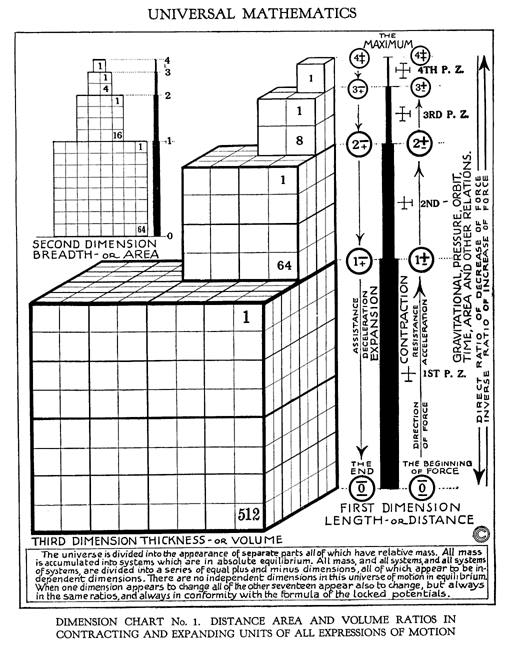# cube ratio

"The reason that each succeeding tonal note, which constitutes the elements of matter, are one octave higher is one entirely of increased pressure. You can better understand that by compressing some air in an enclosed box or tube in which you have inserted a whistle. The more air you pump in, the higher the whistle will sound when you open its valve. If you tighten a wire the same thing will happen. The same harp string can give you many tones if you turn the tuning pins higher, or lower. By the time the first octave string has multiplied in cube ratio nine times, the speed of vibration frequencies and intensity of pressure have reached the incredible proportions of 1,073,741,824 times greater in the 9th string than the first string.

You can better imagine the tremendous explosive pressures which lie behind radium and plutonium bullets by actually seeing the pressure figures. Try it. Start with the first octave at 8. By cubing 8 it equals 64, then 512, then 4,096. The carbon octave comes next with pressure of 32,768. These are your normal pressures, as well as for all organic animal and vegetable life. When you cube your normal pressures you are running into danger. Nitrogen, for example, is phosphorous when cubed once, and it is arsenic when cubed twice. Nitrogen is necessary for you but phosphorous and arsenic will immediately "eat up" the nitrogen of your body and kill you by their radioactive expansion." [Atomic Suicide, page 30-31]Figure 12.09 - Dimensions and Their Relationships

In graphic Figure 12.09 - Dimensions and Relationships it is clear:

Relative Volume

Accumulating Dispersing

4+ = 1/8 of 3+ or 3+ = 8 X 4+ or 81

3+ = 1/8 of 2+ or 2+ = 8 X 3+ or 82

2+ = 1/8 of 1+ or 1+ = 8 X 2+ or 83

Numeric Progressions (units)

1st Dimension = Linear = 1, 2, 4, 8.. (Doubling, nX2)

2nd Dimension = Area = 1, 4, 8, 64.. (Squaring, n2)

3rd Dimension = Volume = 1, 8, 64, 512.. (Cubing, n3)

Volumes

Cube Volume = 1 = 13

Cube Volume = 2 = cube root of 2 = 1.259922 on side

Cube Volume = 4 = cube root of 4 = 1.587403 on side

Cube Volume = 8 = cube root of 8 = 2 on side

therefore

Wavelengths and Frequencies - Octave Relations of Russell's Indig Number System

 Indig Vol. Units Vol. Calc Wavelength Example Octave Note 4 1 13 1 1 cps 4 G as 4th octave 3 8 23 2 1/2 cps 3 F as 3rd octave 2 64 43 4 1/4 cps 2 E as 2nd octave 1 512 83 8 1/8 ccps 1 D as 1st octave 0 C## non-octave

Table 12.02.01 - Wavelengths and Frequencies

Showing linear versus geometric progressions as also other types of progressions (counting methods or scales).